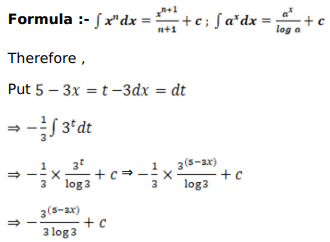# Mark against the correct answer in each of the following:

Question:

Mark $(\sqrt{)}$ against the correct answer in each of the following:

$\int e^{(5-3 x)} d x=?$

A. $\frac{3^{(5-3 x)}}{3(\log 3)}+C$

B. $\frac{3^{(4-3 x)}}{(\log 3)}+C$

C. $-3^{(5-3 x)} \log 3+C$

D. none of these

Solution: